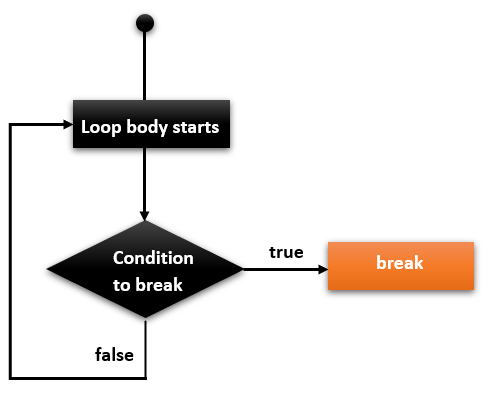# C break Statement

In this tutorial, you will learn how to use break statements in the C programming language with the help of examples.

The break statement allows us to terminate the loop. As soon as the break statement encounters within a loop, the loop iteration stops there immediately and the control goes to the next statement after the loop.

The break keyword is used in both the loops and switch cases. In the case of a nested loop, it stops the execution of the innermost loop and starts executing the next after the code block. The break statement can be used in the following two scenarios.

1. With switch cases
2. With loops

## Syntax

The syntax of the break statement in the C language as follows:

`break;`

## Flowchart of break in C## Example

Following is a simple C program that demonstrates the use of the break statement.

```#include <stdio.h>
int main () {
int a = 1;
while( a < 25 ) {

printf("value of a: %d\n", a);
a++;

if( a > 5) {
/* terminate the loop using break statement */
break;
}
}
printf("Came out of the loop when the value of a: %d",a);
return 0;
}```

### Output

value of a: 1
value of a: 2
value of a: 3
value of a: 4
value of a: 5
Came out of the loop when the value of a: 6

## C break statement with switch cases

You can check the example of the C break statement with switch cases here.

## C break statement with while loop

Check the following example to use the break statement inside the while loop.

```#include<stdio.h>
void main ()
{
int i = 1;
while(i<10)
{
printf("%d\n",i);
i++;
if(i == 5)
break;
}
printf("Came out of while loop");
}```

### Output

1
2
3
4
Came out of while loop

## C break statement with do-while loop

Consider the below example to use the break statement in the do-while loop.

```#include <stdio.h>
int main()
{
// Initialization expression
int i = 1;
do
{
printf("%d\n", i);
i++;
if(i==6){
break;
}
} while (i <= 10);
printf("Came out of do-while loop.");
return 0;
}```

### Output

1
2
3
4
5
Came out of do-while loop.

## C break statement with nested loop

If you use a break statement in the inner loop, it will come out from the inner loop only not from the outer loop.
```#include <stdio.h>
int main()
{
for (int i = 1; i <= 5; i++)
{
for (int j = i; j <= 5; j++)
{
printf("%d\t", j);
if(i==2 && j==2){
break;
} // will break the loop of j only
}
printf("\n");
}
return 0;
}```

### Output

1   2   3   4   5
2
3   4   5
4   5
5